# Glass aquarium

How many m2 of glass are needed to produce an aquarium with bottom dimensions of 70 cm x 40 cm and 50 cm high?

x =  1.38 m2

### Step-by-step explanation: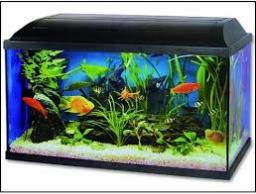Did you find an error or inaccuracy? Feel free to write us. Thank you!Tips to related online calculators
Do you want to convert area units?

#### You need to know the following knowledge to solve this word math problem:

We encourage you to watch this tutorial video on this math problem:

## Related math problems and questions:

• Aquarium II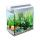Calculate how much glass we need to build an aquarium with a rectangular shape with base 70 cm × 70 cm and a height of 70 cm, if the waste is 2%. Aquarium haven't top glass.
• AquariumThe box-shaped aquarium is 40 cm high; the bottom has dimensions of 70 cm and 50 cm. Simon wanted to create an exciting environment for the fish, so he fixed three pillars to the bottom. They all have the shape of a cuboid with a square base. The base edg
• CardboardHow many m2 of cardboard are needed to make the cuboid with dimensions 40 cm 60 cm and 20 cm?
• AquariumFind how many dm2 of glass we need to make a block-shaped aquarium (the top is not covered) if the dimensions of the aquarium are to be: width 50 cm, length 120 cm, and height 8.5 dm.
• Empty aquariumHow much does an empty aquarium weigh with dimensions: length = 40 cm, width = 30 cm, height = 20 cm, if 1 dm2 of glass weighs 300 g? Calculate its weight in kilograms.
• Cuboid boxHow many m2 paper is needed for the sticking cuboid box of dimensions 50 cm, 40 cm and 30 cm? To the folds add one-tenth the area.
• Insulate houseThe property owner wants to insulate his house. The house has these dimensions 12, and 12 m is 15 m high. The windows have 6 with dimensions 170 and 150 cm. Entrance doors are 250 and 170 cm in size. How many square meters of polystyrene does he need?
• Aquarium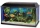I have an aquarium that is 100 cm long and 40 cm wide and 40 cm in height. We fill it with water. How much will it weigh?
• Aquarium 6How high is the water level in the aquarium with a rectangular base 40cm and 50cm if it is filled 0,65hl of water?
• Surface and volumeFind the surface and volume of a cuboid whose dimensions are 1 m, 50 cm, and 6 dm.
• Bathtub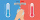Kevin has a bathtub shaped like a block. The bathtub measures 70 cm long, 45 cm wide and 50 cm high. Then the bathtub will be filled with clean water 3/5 of its height. The clean water needed is….
• Surface areaCalculate the surface area of a four-sides 2-m high prism which base is a rectangle with sides 17 cm and 1.3 dm
• Paper boxCalculate whether 11 dm² of paper is sufficient for gluing a box without a lid with bottom dimensions of 2 dm and 15 cm and 12 cm high. Write result as: 0 = No, 1 = Yes
• Concrete tank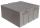How many m3 of concrete is in the tank shape of a cuboid with dimensions of 2000 cm and 5.6 meters and 70 dm if the concrete takes up 3/7 of the tank?
• Triangular prismCalculate a triangular prism if it has a rectangular triangle base with a = 4cm and hypotenuse c = 50mm and height of the prism is 0.12 dm.
• Swimming poolThe swimming pool has the shape of a block with dimensions of 70dm, 25m, 200cm. How many hl of water can fit into the pool?
• Water tankWhat is the height of the cuboid-shaped tank with the bottom dimensions of 80 cm and 50 cm if the 480 liters of water reach 10 cm below the top?